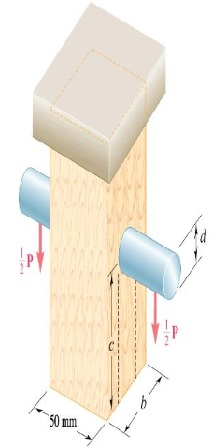A load P is supported as shown by a steel pin that has been inserted in a short wooden member...

Question:

A load P is supported as shown by a steel pin that has been inserted in a short wooden member hanging from the ceiling. The ultiamate strength of the wood used in 61 MPa in tension and 8 Mpa in shear, while the ultimate strength of the steel is 130MPa in shear. Knowing that b=50 mm, c=58 mm, and d=10 mm, determine the load P if an overall factor of safety of 3.3 is desired. (Answer in units of kN)Given data:

• Tension in wood is: {eq}\sigma = 61\;{\rm{MPa}} = 61 \times {10^6}\;{\rm{Pa}}. {/eq}
• Shear stress in wood is: {eq}\tau = 8\;{\rm{MPa}} = 8 \times {10^6}\;{\rm{Pa}}. {/eq}
• Ultimate shear stress in steel is: {eq}{\tau _u} = 130\;{\rm{MPa}} = 130 \times {10^6}\;{\rm{Pa}}. {/eq}
• Side length of wooden member is: {eq}b = 50\;{\rm{mm}} = 0.050\;{\rm{m}}. {/eq}
• Distance of pin from bottom of wooden member is: {eq}c = 58\;{\rm{mm}} = 0.058\;{\rm{m}}. {/eq}
• Diameter of pin is: {eq}d = 10\;{\rm{mm}} = 0.010\;{\rm{m}}. {/eq}
• Factor of safety is: {eq}fos = 3.3. {/eq}

Expression for the cross-sectional area of pin.

{eq}{A_p} = \dfrac{\pi }{4}{d^2} {/eq}

Substitute the values in the above expression.

{eq}\begin{align*} {A_p} &= \dfrac{\pi }{4}{\left( {0.010} \right)^2}\\ &= 7.8598 \times {10^{ - 5}}\;{{\rm{m}}^2} \end{align*} {/eq}

Formula for the stress to determine the load.

{eq}\begin{align*} \dfrac{{{P_1}}}{2} &= {\tau _c}\left( {{A_p}} \right)\\ {P_1} &= 2{\tau _c}\left( {{A_p}} \right) \end{align*} {/eq}

Substitute the values in the above expression.

{eq}\begin{align*} {P_1} &= 2\left( {130 \times {{10}^6}} \right)\left( {7.85398 \times {{10}^{ - 5}}} \right)\\ &= 20420.348\;{\rm{N}} \end{align*} {/eq}

Expression for the area of wooden member affected by tension.

{eq}{A_T} = b\left( {b - d} \right) {/eq}

Substitute the values in the above expression.

{eq}\begin{align*} {A_T} &= 0.050\left( {0.050 - 0.010} \right)\\ &= 0.050\left( {0.04} \right)\\ &= 2 \times {10^{ - 3}}\;{{\rm{m}}^2} \end{align*} {/eq}

Formula for the stress to determine the load.

{eq}{P_1} = \sigma \left( {{A_T}} \right) {/eq}

Substitute the values in the above expression.

{eq}\begin{align*} {P_1} &= \left( {61 \times {{10}^6}} \right)\left( {9.62 \times {{10}^{ - 4}}} \right)\\ &= 122000\;{\rm{N}} \end{align*} {/eq}

Expression for the area of wooden member affected by double shear.

{eq}{A_S} = bc {/eq}

Substitute the values in the above expression.

{eq}\begin{align*} {A_2} &= 0.050\left( {0.058} \right)\\ &= 2.9 \times {10^{ - 3}}\;{{\rm{m}}^2} \end{align*} {/eq}

Formula for the stress to determine the load.

{eq}\begin{align*} \dfrac{{{P_2}}}{2} &= \tau \left( {{A_S}} \right)\\ {P_2} &= 2\tau \left( {{A_S}} \right) \end{align*} {/eq}

Substitute the values in the above expression.

{eq}\begin{align*} {P_2} &= 2\left( {8 \times {{10}^6}} \right)\left( {2.9 \times {{10}^{ - 3}}} \right)\\ &= 46400\;{\rm{N}} \end{align*} {/eq}

{eq}{P_{all}} = \dfrac{{{P_1}}}{{fos}} {/eq}

Here, the load on pin is taken as it has smallest area.

{eq}\begin{align*} {P_{all}} &= \dfrac{{20420.348}}{{3.3}}\\ &= 6187.9842\;{\rm{N}} \end{align*} {/eq}

Thus, the load is 6187.9842 N.